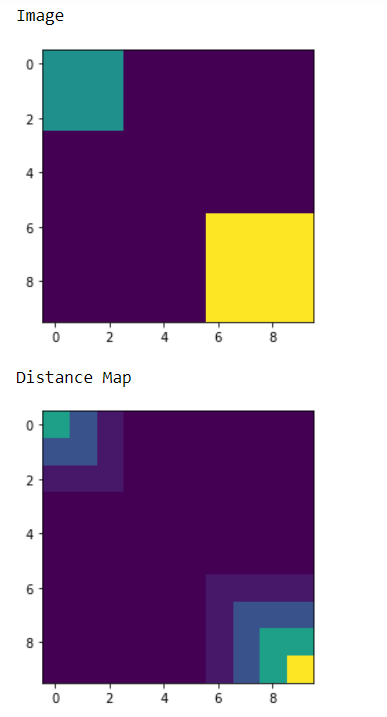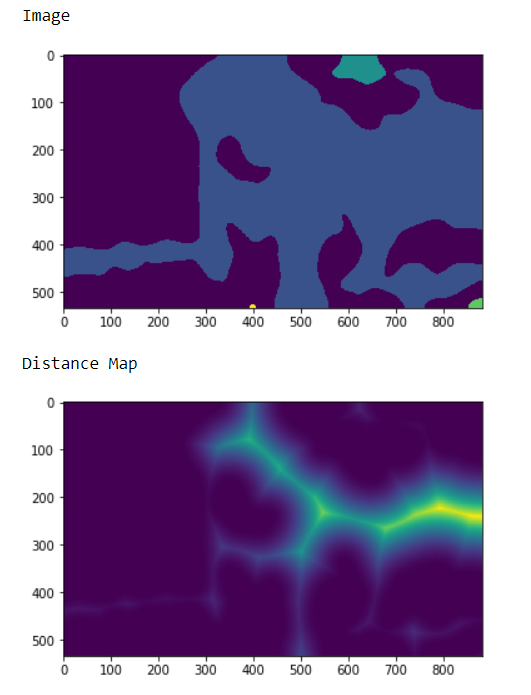# Mahotas – Distance from binary image

In this article we will see how we can obtain distance map of binary image in mahotas. A distance transform, also known as distance map or distance field, is a derived representation of a digital image. The choice of the term depends on the point of view on the object in question: whether the initial image is transformed into another representation, or it is simply endowed with an additional map or field.
In order to do this we will use mahotas.distance method

Syntax : mahotas.distance(img)
Argument : It takes image object which should be binary as argument
Return : It returns image object

Note : Input image should be binary image it can be labeled as well, image should be filtered or should be loaded as grey to make it binary
In order to filter the image we will take the image object which is numpy.ndarray and filter it with the help of indexing, below is the command to do this

`image = image[:, :, 0]`

Example 1:

## Python3

 `# importing required libraries` `import` `mahotas as mh` `import` `numpy as np` `from` `pylab ``import` `imshow, show` ` `  `# creating region` `# numpy.ndarray` `regions ``=` `np.zeros((``10``, ``10``), ``bool``)` ` `  `# setting 1 value to the region` `regions[:``3``, :``3``] ``=` `1` `regions[``6``:, ``6``:] ``=` `1` ` `  `# getting labeled function` `labeled, nr_objects ``=` `mh.label(regions)` ` `  `# showing the image with interpolation = 'nearest'` `print``(``"Image"``)` `imshow(labeled, interpolation ``=``'nearest'``)` `show()`   `# getting distance map` `dmap ``=` `mahotas.distance(labeled)`   `# showing image` `print``(``"Distance Map"``)` `imshow(dmap)` `show()`

Output :Example 2:

## Python3

 `# importing required libraries` `import` `numpy as np` `import` `mahotas` `from` `pylab ``import` `imshow, show` ` `  `# loading image` `img ``=` `mahotas.imread(``'dog_image.png'``)` `   `  `# filtering the image` `img ``=` `img[:, :, ``0``]` `    `  `# setting gaussian filter` `gaussian ``=` `mahotas.gaussian_filter(img, ``15``)` ` `  `# setting threshold value` `gaussian ``=` `(gaussian > gaussian.mean())` ` `  `# creating a labeled image` `labeled, n_nucleus ``=` `mahotas.label(gaussian)` ` `    `print``(``"Image"``)` `# showing the gaussian filter` `imshow(labeled)` `show()`     `# getting distance map` `dmap ``=` `mahotas.distance(labeled)`   `# showing image` `print``(``"Distance Map"``)` `imshow(dmap)` `show()`

Output :Whether you're preparing for your first job interview or aiming to upskill in this ever-evolving tech landscape, GeeksforGeeks Courses are your key to success. We provide top-quality content at affordable prices, all geared towards accelerating your growth in a time-bound manner. Join the millions we've already empowered, and we're here to do the same for you. Don't miss out - check it out now!